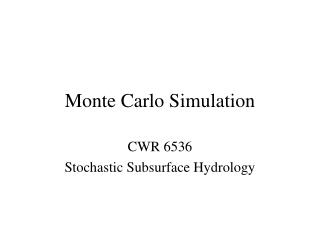DownloadDownload PresentationMonte Carlo Simulation

# Monte Carlo Simulation

Download Presentation## Monte Carlo Simulation

- - - - - - - - - - - - - - - - - - - - - - - - - - - E N D - - - - - - - - - - - - - - - - - - - - - - - - - - -
##### Presentation Transcript

1. Monte Carlo Simulation CWR 6536 Stochastic Subsurface Hydrology

2. Steps in Monte Carlo Simulation • Create input sample space with known distribution, e.g. ensemble of all possible combinations of v, D, q, m values • Run each realization of v, D, q, m values through model to produce output sample space • Repeat experiment many times to get accurate representation of input sample space and accurate statistics of output sample space • Calculate statistics of output sample space, i.e. pdf, mean, variance, etc. as a function of location

3. Steps in Monte Carlo Simulation

4. Primary questions to ask • How to generate input samples? • are random inputs correlated with each other • are random inputs correlated in space or time • How many replicates are required to get reliable output statistics? • test input statistics to be sure they are generated correctly • test convergence of output statistics to constant values • calculate approximate number of replicates needed as get an idea of magnitude of mean and variance of the output.

5. Generating random variables of arbitrary distribution • Generate uniform distribution of random numbers between 0 and 1 (yi) • yi can be considered the cdf of a random variable xi with the arbitrary distribution G(x)

6. Example: Exponential Distribution • Now x is a random variable with cdf G(x) =1-e-ax and pdf ae-ax • Thus can use uniform distribution random number generator to generate random variable of any distribution

7. Generating random fields/processes(See Deutsch & Journel, 1998; Goovaerts, 1997) • Spatially distributed random fields • Categorical indicator simulation to honor specific geometrical patterns (i.e. layering) • Sequential Gaussian simulation, LU decomposition, and/or Turning Bands generator to simulate distribution of continuous properties within geometry • Can generate conditional or unconditional simulations; must specify mean & spatial covariance structure, as well as data to be used for conditioning) • Temporally correlated random processes: • Markov process generators (specify m,s2, transition probabilities)

8. How many replicates are sufficient? • Test input statistics for convergence to known moments • Test output statistics for convergence to constant values • Use confidence intervals to estimate number replicates required to give desired accuracy once have estimate of mean and variance of output

9. 95% Confidence Intervals • Consider the moment estimator • If is normally distributed, a=1.97 std err( )

10. 95% Confidence Intervals • Suppose want 95% confidence intervals to be +/- 10%

11. 95% Confidence Intervals • For the mean • For the std dev